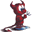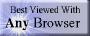music# Horner’s Method

## summary

This subchapter looks at using Horner’s Method to reduce the computational overhead for evaluating a polynomial function.

## free computer programming text book project

If you like the idea of this project,# Horner’s Method

This subchapter looks at using Horner’s Method to reduce the computational overhead for evaluating a polynomial function.

Consider the polynomail function:

ƒ(x) = 8x5 + 5x4 - 3x3 + 9x2 + 2x -7

Assuming that the exponentiation is performed by repeated multiplication (that is, 3x3 is the same as 3 · x · x · x, which is four multiplications), then there are a total of 6 + 4 + 3 + 2 +1 or 15 multiplications and five (5) additions.

We can use Horner’s Method to successively factor out x as follows:

ƒx) = 8x5 + 5x4 - 3x3 + 9x2 + 2x -7
= (8x4 + 5x3 - 3x2 + 9x + 2)x -7
= ((8x3 + 5x2 - 3x + 9)x + 2)x -7
= (((8x2 + 5x - 3)x + 9)x + 2)x -7
= ((((8x + 5)x - 3)x + 9)x + 2)x -7

The new form of the polynomial only requires five multiplcations and five additions,.

This is an improvement from N(N +1)/2 multiplications and N additions to N multiplications and N additions.

We can use a simple loop to do the steps in this process.

## Pascal

{numbers will be entered in the order x, -7, 2, 9, -3, 5, 8}
writeln('enter x: ');
lower := 1;
upper := 5;
subtotal := 0;
writeln('enter coefficients right to left:');
for counter := lower to higher
do begin
writeln('enter next coefficient ');
subtotal := subtotal * x + n;
end;   {for}

Note that we can slightly increase efficiency by moving the first coefficient out of the loop (because we don’t really need to multiply by zero):

## Pascal

{numbers will be entered in the order x, -7, 2, 9, -3, 5, 8}
writeln('enter x: ');
lower := 2;
upper := 5;
writeln('enter coefficients right to left:');
writeln('enter first coefficient ');
for counter := lower to higher
do begin
writeln('enter next coefficient ');
subtotal := subtotal * x + n;
end;   {for}

It should be easy to write a short program that can evaluate a polynomial of any degree. And that is left as a problem for the student.

The next refinement to our sample code is to get the information from arrays rather than from user input.

## Pascal

{array has the numbers in the order x, -7, 2, 9, -3, 5, 8}
{x is set to the value to be evaluated}
lower := 2;
upper := 5;
subtotal := n;
for counter := lower to higher
do begin
writeln('enter next coefficient ');
subtotal := subtotal * x + n[counter];
end;   {for}

# free music player coding example

Coding example: I am making heavily documented and explained open source code for a method to play music for free — almost any song, no subscription fees, no download costs, no advertisements, all completely legal. This is done by building a front-end to YouTube (which checks the copyright permissions for you).

View music player in action: www.musicinpublic.com/.

Create your own copy from the original source code/ (presented for learning programming).

## view text bookHTML file

Because I no longer have the computer and software to make PDFs, the book is available as an HTML file, which you can convert into a PDF.

 Tweets by @osdata

## free computer programming text book project

Building a free downloadable text book on computer programming for university, college, community college, and high school classes in computer programming.

If you like the idea of this project,

send donations to:
Milo
PO Box 1361
Tustin, California 92781

Supporting the entire project:

If you have a business or organization that can support the entire cost of this project, please contact Pr Ntr Kmt (my church)

Some or all of the material on this web page appears in theThis web site handcrafted on Macintoshcomputers using Tom Bender’s Tex-Edit Plusand served using FreeBSD.†UNIX used as a generic term unless specifically used as a trademark (such as in the phrase “UNIX certified”). UNIX is a registered trademark in the United States and other countries, licensed exclusively through X/Open Company Ltd.

Names and logos of various OSs are trademarks of their respective owners.